# Minimum Curvature Method

The Minimum Curvature Method smooths two straight-line segments of the Balanced Tangential Method by using the Ratio Factor (RF).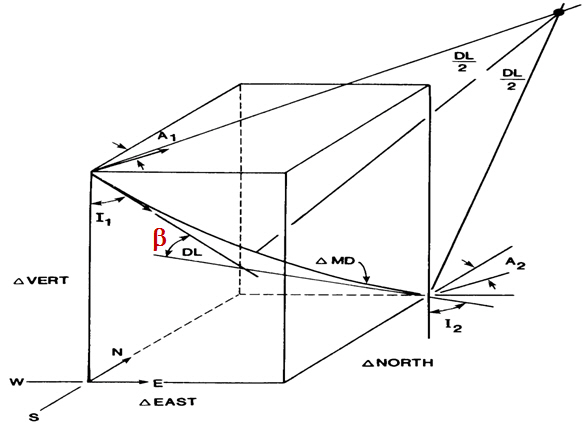The formulas for the Minimum Curvature Method are listed below;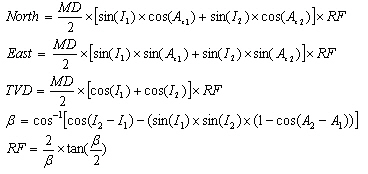Where;
MD = Measured Depth between surveys in ft
I1 = Inclination (angle) of upper survey in degrees
I2 = Inclination (angle) of lower in degrees
Az1= Azimuth direction of upper survey
Az2 = Azimuth direction of lower survey
RF = Ratio Factor
ß  is the dog leg angle.

The following example is the Minimum Curvature Method Calculation

Survey 1
Depth = 3500 ft
Inclination = 15 degree (I1)
Azimuth = 20degree (Az1)

Survey 2
Depth = 3600 ft
Inclination = 25 degree (I2)
Azimuth = 45 degree (Az2)

Solution:
MD = 3600 – 3500 = 100 ft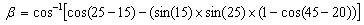ß  = 0.22605 radian = 12.95 degreeRF= 1.00408

As per the formulas above, you will get answers as listed below;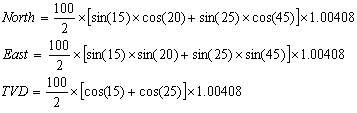North = 27.22 ft
East = 19.45 ft
TVD = 94.01 ft

Please find the Excel sheet used for The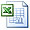Minimum Curvature Method Calculation

Share the joyWorking in the oil field and loving to share knowledge.

### 6 Responses to Minimum Curvature Method

1.deyaa says:

very simple and very cool, thanks

2.Mr. Phillip Sand Hansel II says:

In the Minimum Curvature calculation of the Ratio Factor, Beta is shown in radians on the left, but in degrees on the right. Below the initial formula it mentions that “Beta must be in radians”. Is the equation valid with mixed units for Beta?

3.Mr. Phillip Sand Hansel II says:

I know it is bad form, but I’ve answered my own question; the solution for RF incorrectly shows degrees where it should show radians (in order to get the calculation to match the results). The “excel solution” spreadsheet is nice, but since it is locked, one cannot view the entire formula. This required extra effort to determine how the equation should be rendered in excel. I did eventually get it to work, so I am grateful for the example.

4.Rikie says:

5.Steve says: